# Stereo Matching文献笔记之（八）：《On Building an Accurate Stereo Matching System on Graphics Hardware》读后感~

(转载请注明：http://blog.csdn.net/wsj998689aa/article/details/49403353, 作者：迷雾forest)

## 算法详解

### 2. 特征融合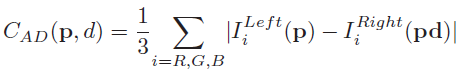int hei = lImg.rows;
int wid = lImg.cols;
Mat lGray, rGray;
Mat tmp;
lImg.convertTo( tmp, CV_32F );
cvtColor( tmp, lGray, CV_RGB2GRAY );
lGray.convertTo( lGray, CV_8U, 255 );
rImg.convertTo( tmp, CV_32F );
cvtColor( tmp, rGray, CV_RGB2GRAY );
rGray.convertTo( rGray, CV_8U, 255 );

// prepare binary code
int H_WD = CENCUS_WND / 2;
bitset<CENCUS_BIT>* lCode = new bitset<CENCUS_BIT>[ wid * hei ];
bitset<CENCUS_BIT>* rCode = new bitset<CENCUS_BIT>[ wid * hei ];
bitset<CENCUS_BIT>* pLCode = NULL;
bitset<CENCUS_BIT>* pRCode = NULL;

// 代价计算
// 计算左图
for(int i = 0; i < reflect_pts_num; i++)
{
int repeated = reflect[i].repeated;
if(repeated == 1)
{
continue;
}

int x     = reflect[i].x;
int y     = reflect[i].y;
int index = reflect[i].index;

uchar pLData = lGray.at<uchar>( y, x );
pLCode       = &(lCode[index]);

int bitCnt = 0;
for( int wy = - H_WD; wy <= H_WD; wy ++ )
{
int qy = ( y + wy + hei ) % hei;
for( int wx = - H_WD; wx <= H_WD; wx ++ )
{
if( wy != 0 || wx != 0 )
{
int qx = ( x + wx + wid ) % wid;
uchar qLData = lGray.at<uchar>( qy, qx );
( *pLCode )[bitCnt] = ( pLData > qLData );
bitCnt ++;
}
}
}
}

// 计算右图
pRCode = rCode;
for( int y = 0; y < hei; y ++ ) {
uchar* pRData = ( uchar* ) ( rGray.ptr<uchar>( y ) );
for( int x = 0; x < wid; x ++ ) {
int bitCnt = 0;
for( int wy = - H_WD; wy <= H_WD; wy ++ ) {
int qy = ( y + wy + hei ) % hei;
uchar* qRData = ( uchar* ) ( rGray.ptr<uchar>( qy ) );
for( int wx = - H_WD; wx <= H_WD; wx ++ ) {
if( wy != 0 || wx != 0 ) {
int qx = ( x + wx + wid ) % wid;
( *pRCode )[ bitCnt ] = ( pRData[ x ] > qRData[ qx ] );
bitCnt ++;
}
}
}
pRCode ++;
}
}

// 代价体计算
bitset<CENCUS_BIT> lB;
bitset<CENCUS_BIT> rB;
for(int i = 0; i < reflect_pts_num; i++)
{
int repeated = reflect[i].repeated;
if(repeated == 1)
{
continue;
}

int x = reflect[i].x;
int y = reflect[i].y;
int index = reflect[i].index;

lB = lCode[index];
for(int d = 0; d < maxDis; d ++)
{
if(x - d >= 0)
{
rB = rCode[ index - d ];
costVol[d].at<double>(y, x) = ( lB ^ rB ).count();
}
else
{
costVol[d].at<double>(y, x) = CENCUS_BIT;
}
}
}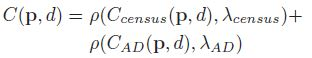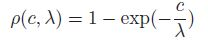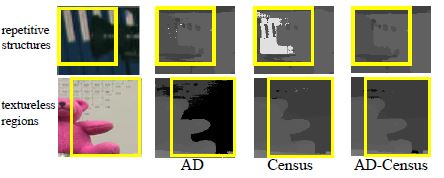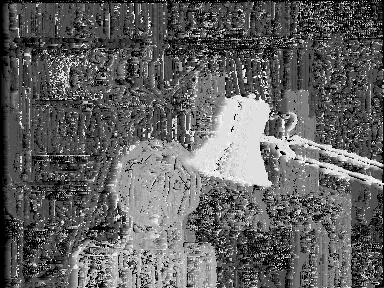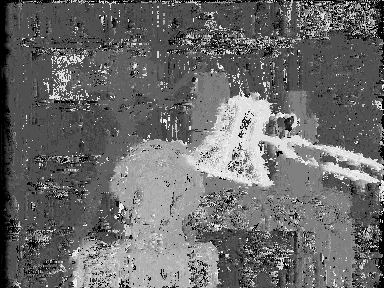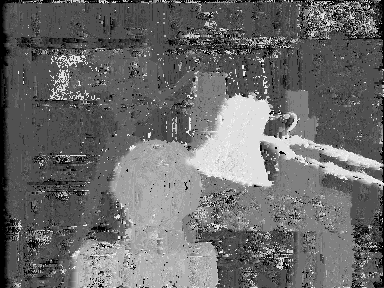### 3. 自适应区域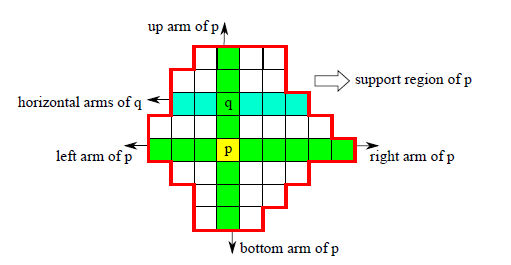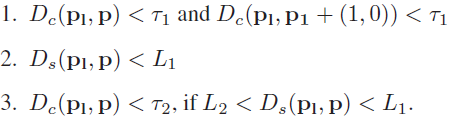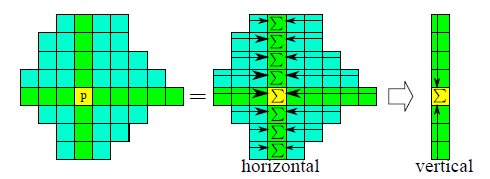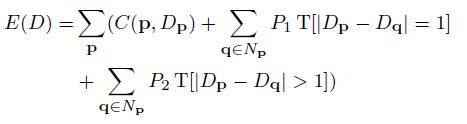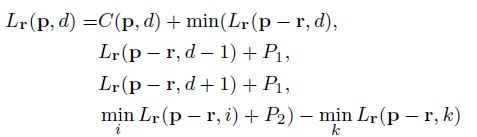## 后续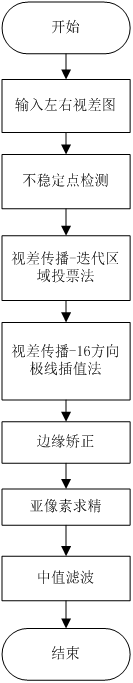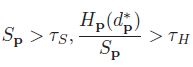## 总结

1. 自适应区域确实有用，没有了它视差图效果就会大打折扣，所谓正途。

2. 自适应区域是为了发表论文才加上去的，如果没有这一块，论文的表面含金量就缩水了，所谓鸡肋。

3. 自适应区域的真实目的是为了后续的迭代区域求精，所以索性也用于代价聚合，所谓醉翁之意不在酒。©️2019 CSDN 皮肤主题: 大白 设计师: CSDN官方博客